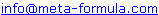# Metastock Indicators Formula Click here to go back to Metastock Formula Index

Before you begin, if you haven't read the "The Search for the Holy Grail
& the Perfect Indicator" click here and scroll halfway down the page to
view it now.

Metastock step-by-step.

ADX Raw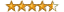Bang For The Buck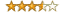Coppock Indicator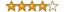Darvis Box - Based on "How I made 2 million on the stockmarket"
Dr Elder's Force Index

Elliot Wave Identification

Fibonacci Trader- Fixed Balance Point- REVISED
Gann High Low

Gann-Swing
Gann-Trend
Guppy MMA OscillatorHighest High
MACD Histogram
MA oscillator, Sine-weightedMetastock Automatic Trendline FormulaModified Williams %R Metastock Indicator
Psychology IndexSlope of a Linear Regression Line
Stochastic Momentum IndicatorResistence & Support
Trailing Stoploss ATR – Long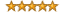True Strength Index
Zero Lag EMA
Zero Lag MACD

ADX Raw - Metastock Indicator FormulaBy Equis International Metastock Indicators
and published in the Oct99 TASC

Periods:= Input("Enter time periods",1,100,14);

PlusDM:= If(HIGH>Ref(HIGH,-1) AND
LOW>=Ref(LOW,-1), HIGH-Ref(HIGH,-1),
If(HIGH>Ref(HIGH,-1) AND LOW<Ref(LOW,-1)
AND HIGH-Ref(HIGH,-1)>Ref(LOW,-1)-LOW,
HIGH-Ref(HIGH,-1), 0));
DIPlus:= 100 * Wilders(PlusDM,Periods) /
ATR(Periods);

MinusDM:= If(LOW<Ref(LOW,-1) AND
HIGH<=Ref(HIGH,-1), Ref(LOW,-1)-LOW,
If(HIGH>Ref(HIGH,-1) AND LOW<Ref(LOW,-1)
AND HIGH-Ref(HIGH,-1)<Ref(LOW,-1)-LOW,
Ref(LOW,-1)-LOW, 0));
DIMinus:= 100 * Wilders(MinusDM,Periods) /
ATR(Periods);

DIDif:= Abs(DIPlus - DIMinus);
DISum:= DIPlus + DIMinus;

Bang For The Buck - Metastock Indicator Formulaby MetaStock Programming Study Guide

This indicator shows the possible dollar return (on a \$10,000 account)
for a security on any given period. This is calculated by dividing a
\$10,000 account by the closing price. This number is then multiplied by
the average range of the security for the last 200 periods. The
interpretation is such that the higher the value, the higher the profit
potential.

((10000/C)* (Mov(ATR(1),200,S))/100)

Coppock Indicator - Metastock Indicator Formulaby MetaStock Programming Study Guide

The Coppock Indicator was developed by Edwin Coppock, a US
investment advisor. It is a momentum oscillator that was designed for
long term investors to begin accumulation at the beginning of a bull
market. The Coppock Indicator signals the beginning of a bull market
when it crosses above the zero line. This signal is usually after the first
leg of a bull market is underway, thus it’s highly reliable.

Mov(ROC(C,14,%)+ROC(C,11,%),10,W);0

Darvis Box - Based on "How I made 2 million on the stockmarket"
Jason Prestwidge

Periods:=Input("periods",1,260,260);

Topbox:=If(Ref(H,-3)>=Ref(HHV(H,Periods),-4) AND Ref(H,-2)<
Ref(H,- 3) AND Ref(H,-1)<Ref(H,-3) AND H< Ref(H,-3),
Ref(H,-3),PREVIOUS);

Botbox:=If(Ref(H,-3)>=Ref(HHV(H,Periods),-4) AND Ref(H,-2)
<Ref(H,-3) AND Ref(H,-1)<Ref(H,-3) AND H<
Ref(H,-3),LLV(L,4),PREVIOUS);

Topbox;
Botbox;

Dr Elder's Force Index - Metastock Indicator Formula
by MetaStock Programming Study Guide

FI:=(C-Ref(C,-1))*V;Mov(FI,13,E)
{Smoothed by 13 period exponential moving average}

Dr Elder also refers to a 2 period Force Index in which case, you
would change the ‘13’ above to ‘2’.

(The following explanatory notes are taken from ‘Trading for a Living’
by Dr. Alexander Elder, Published by John Wiley & Sons, Inc, 1993)

“Force Index is an oscillator developed by this author. It measures
the force of bulls behind every rally and of bears behind every decline.

Force Index combines three essential pieces of market information –
the direction of price change, its extent, and trading volume.
It provides a new, practical way of using volume to make trading
decisions.

Force Index can be used raw, but it works better if you smooth it
with a moving average. Force Index smoothed with a short MA
helps pinpoint entry and exit points. Force Index smoothed with
a long MA reveals major changes in the force of bulls and bears.

A 2-day EMA of Force Index provides a minimal degree of smoothing.
It is useful for finding entry points into the markets. It pays to buy
when the 2-day EMA is negative and sell when it is positive, as long
as you trade in the direction of the 13-day EMA of prices.

A 13-day EMA of Force Index tracks longer term changes in the force
of bulls and bears. When it crosses above its centerline, it shows the
bulls are in control. When it turns negative, it shows that bears are in
control. Divergences between 13-day EMA of Force Index and prices
identify important turning points.”

Elliot Wave Identification
- Metastock Indicator Formula
by Kevin Campbell

As far as using MetaStock for identifying waves, use a 5/34 histogram
for finding wave 4, the end of wave 3 and for help with identifying
wave 1/2, which apparently Advanced Get uses extensively. You can
write MetaStock explorations/templates/experts, etc., with this
indicator; e.g., explorations to find the peaks and troughs of the
5/34 histogram.

The version of the indicator I use in MetaStock v6.52 is:

Mov(OscP(5,34,E,\$),5,S)

-150 days minimum of data.

The peaks of the histogram help identify waves 1, 3 and 5 and troughs
for waves 2 and 4. Use MetaStock line studies (both trendlines,
channels and fib retracements) for additional wave
identification/analysis. Of course, you can label the waves with the
text box.

- Metastock Indicator Formula

{Fixed Balance Point Calculation}
FBC:=If(Mc1=0 AND Fc1>2,
{then}(Ref(HHV(H,LastValue(mc1)),-1)+
Ref(LLV(L,LastValue(Mc1)),-1)+
Ref(C,-1))/3,
{else}If(Fc1=0 AND Mc1>5,
{then}(HHV(H,LastValue(Fc2))+
LLV(L,LastValue(Fc2))+C)/3,
{else}If(Fc1=0,
{then}(HHV(H,LastValue(Mc1))+
LLV(L,LastValue(Mc1))+C)/3,
{else}0)));
{Fixed Balance Point Plot}
FBP:=ValueWhen(1,FBC>0,FBC);
FBP;

- Metastock Indicator Formula

dt:=DayOfWeek();
DBC:=(HighestSince(5,DayOfWeek()=dt,H)+
LowestSince(5,DayOfWeek()=dt,L)+CLOSE)/3;
DBC

Gann High Low - Metastock Indicator Formula

{name: GANN-HiLo}

HLd:=If(CLOSE>Ref(Mov(H,3,S),-1),
{then}1,
{else}If(CLOSE<Ref(Mov(L,3,S),-1),
{then}-1,
{else}0));
HLv:=ValueWhen(1,HLd<>0,HLd);
HiLo:=If(HLv=-1,
{then}Mov(H,3,S),
{else}Mov(L,3,S));
HiLo;

Gann-Swing - Metastock Indicator Formula

{Market swing is defined as:
Up = 2 higher highs,
Down = 2 lower highs.}
Us:=BarsSince((H > Ref(H,-1)) AND (Ref(H,-1) >
Ref(H,-2)));
Ds:=BarsSince((L < Ref(L,-1)) AND (Ref(L,-1) <
Ref(L,-2)));
Sd1:=If(Us=0,
{then}If(Ref(L,-1)<>LowestSince(1,Ds=0,L),
{then}1,
{else}0),
{else}If(Ds=0,
{then}If(Ref(H,-1)<>
HighestSince(1,Us=0,H),
{then}-1,
{else}0),
{else}0));
Sd2:=If(Sd1=1,
{then}1,
{else}0),
{else} If(Sd1=-1,
{then}-1,
{else}0),
{else}0));
TD1:=ValueWhen(1,Sd2<>0,Sd2);
Td1;

Gann-Trend - Metastock Indicator Formula

{Swing Direction}
Sd:= FmlVar("GANN-Swing","TD1") ;
{Swing Change High}
Sch:=If(Sd=1 AND Ref(sd,-1)=-1,
{then}1,
{else}0);
{Swing Change Low}
Scl:=If(Sd=-1 AND Ref(Sd,-1)=1,
{then}1,
{else}0);
{Peak Value}
Pv:=If(Scl=1,
{then}HighestSince(1,Sch=1,H),
{else}0);
{Trough Value}
Tv:=If(Sch=1,
{then}LowestSince(1,Scl=1,L),
{else}0);
{Trend Direction}
Td:=If(H>ValueWhen(1,Pv>0,Pv),
{then}1,
{else}If(L<ValueWhen(1,Tv>0,Tv),
{then}-1,
{else}0));
{UpTrend=1 DownTrend =-1}
Tdv:=ValueWhen(1,Td<>0,Td);
Tdv;

Guppy MMA Oscillator - Metastock Indicator Formulaby Leon Wilson

Indicator Name:- GMMACD \$

Trigger:=Input("Trigger Line",1,55,21);

SHORT:=(Mov(CLOSE,3,E)+Mov(CLOSE,5,E)+
Mov(CLOSE,8,E)+Mov(CLOSE,10,E)+
Mov(CLOSE,12,E)+Mov(CLOSE,15,E));
LONG:=(Mov(CLOSE,30,E)+Mov(CLOSE,35,E)+
Mov(CLOSE,40,E)+Mov(CLOSE,45,E)+
Mov(CLOSE,50,E)+Mov(CLOSE,60,E));

Short-Long;
Mov(Short-Long,Trigger,E);
0;

Indicator Name:- GMMACD %

Trigger:=Input("Trigger Line",1,55,21);

SHORT:=(Mov(CLOSE,3,E)+Mov(CLOSE,5,E)+
Mov(CLOSE,8,E)+Mov(CLOSE,10,E)+
Mov(CLOSE,12,E)+Mov(CLOSE,15,E));
LONG:=(Mov(CLOSE,30,E)+Mov(CLOSE,35,E)+
Mov(CLOSE,40,E)+Mov(CLOSE,45,E)+
Mov(CLOSE,50,E)+Mov(CLOSE,60,E));

((Short-Long)/Long)*100;
Mov(((Short-Long)/Long)*100,Trigger,E);
0;

Highest High - Metastock Indicator Formula
By MetaStock Programming Study Guide

periods := Input("Enter the number of periods for the highest
high", 1, 9999, 120) ; C>Ref(HHV(C, periods ),-1)

MACD Histogram - Metastock Indicator Formula
By MetaStock Programming Study Guide

MACD()-Mov(MACD(),9,E);0

MA oscillator, Sine-weighted
By Jose Silva

{ Normalized, lag-less, Sine-weighted
Mov Avg & MA Oscillator v1.0 }
{ Divergence signals between SWMA & Oscillator:
+1=Long, -1=Short }
{ http://users.bigpond.com/prominex/pegasus.htm }

plot:=Input("-SWMA Osc, -SW Mov Avg,
-Divergences",1,3,1);
pds:=Input("normalizing periods (1=none)",
1,2520,252);

SD:=30 {180/12};
S1:=Sin(1*SD)*C;
S2:=Sin(2*SD)*Ref(C,-1);
S3:=Sin(3*SD)*Ref(C,-2);
S4:=Sin(4*SD)*Ref(C,-3);
S5:=Sin(5*SD)*Ref(C,-4);
S6:=Sin(6*SD)*Ref(C,-5);
S7:=Sin(7*SD)*Ref(C,-6);
S8:=Sin(8*SD)*Ref(C,-7);
S9:=Sin(9*SD)*Ref(C,-8);
S10:=Sin(10*SD)*Ref(C,-9);
S11:=Sin(11*SD)*Ref(C,-10);

den:=
Sin(SD)+Sin(2*SD)+Sin(3*SD)+Sin(4*SD)+Sin(5*SD);
SWMA:=(S1+S2+S3+S4+S5)/den;
den:=Sin(SD)+Sin(2*SD)+Sin(3*SD)+Sin(4*SD)
+Sin(5*SD)+Sin(6*SD)+Sin(7*SD)+Sin(8*SD)
+Sin(9*SD)+Sin(10*SD)+Sin(11*SD);
SWosc:=(S1+S2+S3+S4+S5+S6+S7+S8+S9+S10+S11)/den;
SWoscNorm:=(SWosc-LLV(SWosc,pds))
/(HHV(SWosc,pds)-LLV(SWosc,pds)+.000001)*100;
SWoscNorm:=If(pds<2,SWosc,SWoscNorm);
up:=SWoscNorm>Ref(SWoscNorm,-1)
AND SWMA<Ref(SWMA,-1);
dw:=SWoscNorm<Ref(SWoscNorm,-1)
AND SWMA>Ref(SWMA,-1);

If(plot=1,SWoscNorm,If(plot=2,SWMA,up+-dw))

Metastock Automatic Trendline Formula -
Metastock Indicator FormulaMike Helmacy www.techanalysis.com

This formula will draw a trendline from the most recent bottom.
The L (low) can be changed to C (close) and the 10 can be
changed to a different percent value. You will also need to change
the line style to the last one in the drop down list.

Trough(1,L,10)+ ((((Trough(1,L,10) - Trough(2,L,10)) /
(TroughBars(2,L,10)-TroughBars(1,L,10))) *
TroughBars(1,L,10)))

Modified Williams %R Metastock Indicator -
Metastock Indicator Formula

by Rajat Bose

{All I have done here is to substitute High and Low of any bar with
that of Bollinger Band Top and Bollinger Band Bottom. I have tested
it on various time periods (for Bollinger Bands) using 2 standard
deviations. It sometimes gives an early indication of reversals than
the Williams %R of the same period. Divergences have also been
somewhat better. However, the structure shows that most of its
properties are similar to those of the Williams %R or, for that matter
, of any other overbought-oversold indicator.}

Periods := Input("Time Period", 3,50,5);
NumDev := Input("No. of Standard Deviations", 1,5,2);

(100*(C-BBandBot(C, Periods, S, NumDev))/((( BBandTop(C,
Periods, S, NumDev))-(BBandBot(C, Periods, S, NumDev)))))

Psychology Index -
Metastock Indicator Formulaby Glenn Wallace
- Futures Magazine, Vol.29 No.6, June 2000, P.48

There was an overbought/oversold indicator described in the June
2000 Futures Magazine called the Psychological Index. It looked sort
of interesting, so I wrote the MetaStock code for it:

LookBack:= Input("Number of lookback periods", 2, 100, 12);
UThreshold:= Input("Upper threshold (%)", 0, 100, 75);
LThreshold:= Input("Lower threshold (%)", 0, 100, 25);
UpDay:= If(CLOSE > Ref(CLOSE,-1), 1, 0);
PsychIndex:= Sum(UpDay,LookBack) / LookBack * 100;
PsychIndex; UThreshold; LThreshold

Resistance and Support - Metastock Indicator Formula

LookBack := Input("Look Back Periods",1,1000,10);
Resistance :=ValueWhen(1,Cross(Mov(C, LookBack,
S),C),HHV(H, LookBack));
Support :=ValueWhen(1,Cross(C,Mov(C, LookBack,
S)),LLV(L, LookBack));
Resistance;
Support;

Slope of a Linear Regression Line - Metastock Indicator Formula

The following custom formula will return the slope of a
Linear Regression Line

tp:=Input("Time Periods",1,200,21);
((tp*(Sum(Cum(1)*C,tp)))-(Sum(Cum(1),tp)*(Sum(C,tp))))/
((tp*Sum(Pwr(Cum(1),2),tp))- Pwr(Sum(Cum(1),tp),2))

Stochastic Momentum Indicator - Metastock Indicator Formula
January 1993 issue of Stocks & Commodities magazine100 * ( Mov( Mov(C - (.5 * ( HHV(H,13) + LLV(L,13))),25,E),2,E)
/ (.5*Mov( Mov( HHV(H,13) - LLV(L,13),25,E),2,E)))

Trailing Stoploss ATR – Long - Metastock Indicator Formula
By MetaStock Programming Study GuideThis ATR based indicator is ideal for existing long trades. When
plotted on a chart we will be prompted for the trade entry date
and the multiple of ATR we wish to use as the trailing exit.

PDay := Input("Day of Month" ,1,31,1);
PMonth := Input("Month" ,1,12,1);
PYear := Input("Year",2000,2010,2002);
PATR := Input("Multiple of ATR",1,10,2);
TSE1 := BarsSince(DayOfMonth() = PDay AND Month() = PMonth
AND Year() = PYear);
TSE2 := HighestSince(1,TSE1=0,H ) ;
TSE3 := TSE2 - PATR*ATR(15);

HighestSince(1 ,TSE1 = 1 ,TSE3 )

True Strength Index - Metastock Indicator Formula
January 1993 issue of Technical Analysis of Stocks and Commodities.

100 * ( Mov( Mov( ROC(C,1,\$),25,E),13,E) / Mov( Mov(
Abs( ROC(C,1,\$)),25,E),13,E))

Zero Lag EMA
- Metastock Indicator Formula
by Peter Martin [email protected]

Here's my Metastock 6.2 coded version of the Zero Lag Moving
Average, as described in the April, 2000, issue of
Technical Analysis of Stocks and Commodities. I've also used
it to construct a Zero Lag MACD and a Zero Lag MACD trigger
signal.

Period:= Input("What Period",1,250,10);
EMA1:= Mov(CLOSE,Period,E);
EMA2:= Mov(EMA1,Period,E);
Difference:= EMA1 - EMA2;
ZeroLagEMA:= EMA1 + Difference;
ZeroLagEMA

Zero Lag MACD - Metastock Indicator Formula
by Peter Martin [email protected]

EMA1:= Mov(CLOSE,13,E);
EMA2:= Mov(EMA1,13,E);
Difference:= EMA1 - EMA2;
ZeroLagEMA13:= EMA1 + Difference;
EMA1:= Mov(CLOSE,21,E);
EMA2:= Mov(EMA1,21,E);
Difference:= EMA1 - EMA2;
ZeroLagEMA21:= EMA1 + Difference;
ZeroLagMACD:=ZeroLagEMA13 - ZeroLagEMA21;
ZeroLagMACD

Please email to